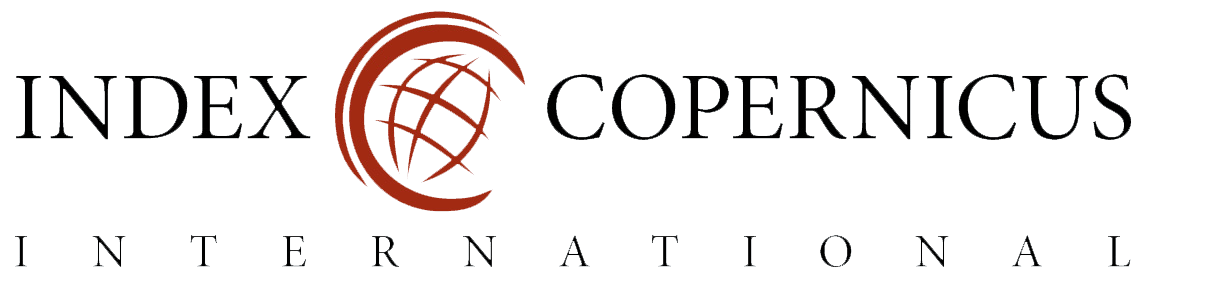Mathematical Modeling and Computing

State Registration Certificate (Ukraine): КВ №24009-13849Р (2014--2018: КВ №21679-1579Р)

The journal is published four times a year (2014--2020: twice a year).

"Mathematical Modeling and Computing" is included in"Mathematical Modeling and Computing" is included in the list of professional editions of Ukraine (category "A") in the following fields: physics and mathematics sciences, technical sciences (104, 105, 111, 113, 121, 122, 124, 126).

#### History of foundation

"Mathematical Modeling and Computing" (or "MMC", or "Math. Model. Comput.") was founded in 2014. And its first issue was published in October of 2014. Founders of the MMC were Lviv Polytechnic National University and Center of Mathematical Modelling of IAPMM (Ukraine).

#### Focus

The journal contains researches and reviews on the following issues in the field of mathematical modeling and computing:

• mathematical modeling non-equilibrium and nonlinear processes;
• mathematical modeling and simulation of mass transfer processes;
• mathematical models of interrelated fields;
• mathematical models in deformable solids;
• numerical methods and software for problems of mathematical mode­ling.